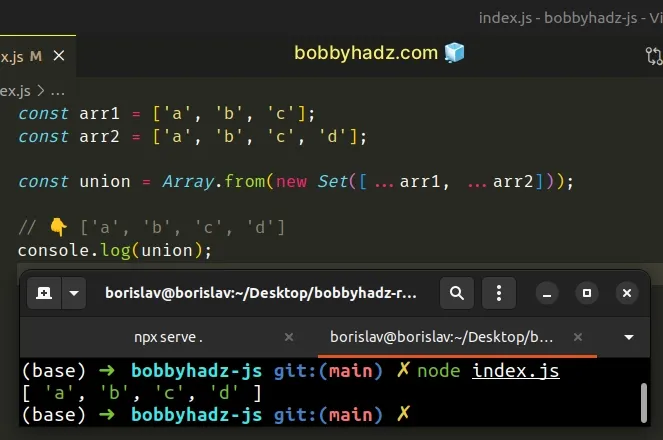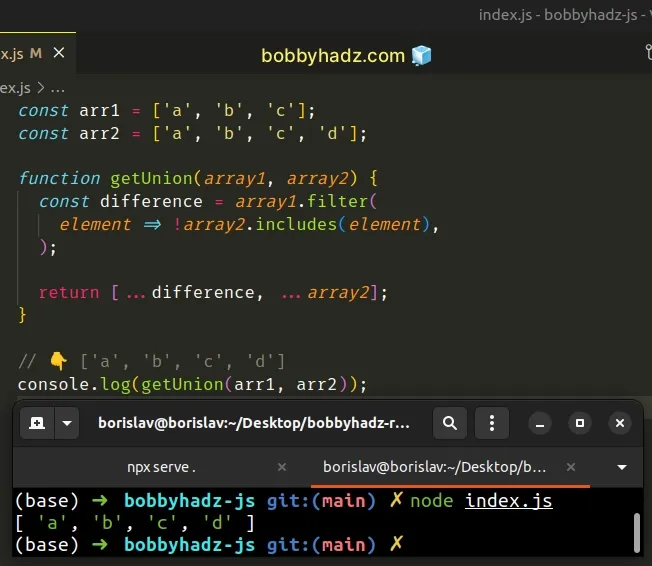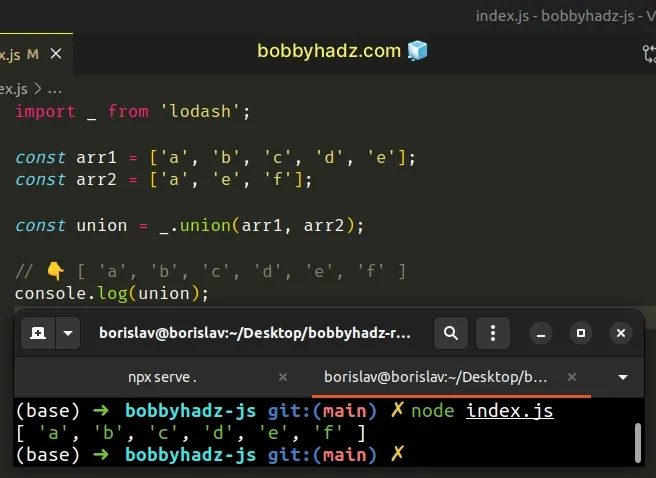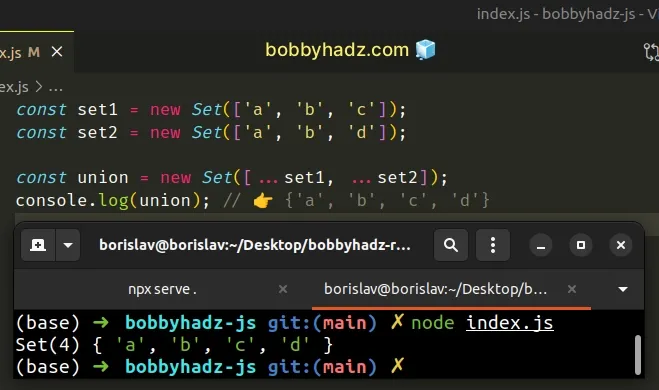# Get the Union of Two Arrays or Sets in JavaScriptLast updated: Dec 27, 2022
5 min## #Get the Union of Two Arrays in JavaScript

To get a union of two arrays:

1. Use the spread syntax (...) to merge the arrays into a third array.
2. Pass the third array as a parameter to the `Set()` constructor to remove the duplicates.
3. Convert the `Set` back to an array.
index.js
```Copied!```const arr1 = ['a', 'b', 'c'];
const arr2 = ['a', 'b', 'c', 'd'];

const union = Array.from(new Set([...arr1, ...arr2]));

// 👇️ ['a', 'b', 'c', 'd']
console.log(union);
``````The union of two `Set` objects is the smallest `Set` which contains all the elements of both `Set` objects.

We used the Set() constructor to remove all of the duplicates from the two arrays.

`Set` objects only store unique values, so all of the duplicates get automatically removed.

If we pass it an array containing the same value multiple times to the `Set()` constructor, it would only get added once to the `Set`.

index.js
```Copied!```console.log(new Set(['a', 'a', 'a'])); // 👉️ { 'a' }
``````

We used the spread syntax (...) to unpack the values of the two arrays into a third array.

index.js
```Copied!```const arr1 = ['a', 'b', 'c'];
const arr2 = ['a', 'b', 'c', 'd'];

const union = Array.from(new Set([...arr1, ...arr2]));

// 👇️ ['a', 'b', 'c', 'd']
console.log(union);
``````

The last step is to convert the set back to an array, using the Array.from method.

You can also use the spread syntax (...) to convert the `Set` object to an array, but it makes the code more difficult to read.

index.js
```Copied!```const arr1 = ['a', 'b', 'c'];
const arr2 = ['a', 'b', 'c', 'd'];

const union = [...new Set([...arr1, ...arr2])];

// 👇️ ['a', 'b', 'c', 'd']
console.log(union);
``````

Alternatively, you can use the `Array.filter()` method.

## #Get the Union of Two Arrays using `Array.filter()`

This is a two-step process:

1. Get the elements that are contained in the first array and not contained in the second array.
2. Merge the result with the elements of the second array.
index.js
```Copied!```const arr1 = ['a', 'b', 'c'];
const arr2 = ['a', 'b', 'c', 'd'];

function getUnion(array1, array2) {
const difference = array1.filter(
element => !array2.includes(element)
);

return [...difference, ...array2];
}

// 👇️ ['a', 'b', 'c', 'd']
console.log(getUnion(arr1, arr2));
``````We used the Array.filter method to iterate over the first array.

On each iteration, we check if the current element is not contained in the second array.

The output of the `filter()` method is a new array that contains the elements of the first array that are not in the second array.

index.js
```Copied!```const arr1 = ['a', 'b', 'c', 'd', 'e', 'f'];
const arr2 = ['a', 'b', 'c', 'd'];

const difference = arr1.filter(element => !arr2.includes(element));

console.log(difference); // 👉️ [ 'e', 'f' ]
``````

The last step is to merge the difference with the values of the second array.

index.js
```Copied!```function getUnion(array1, array2) {
const difference = array1.filter(element => !array2.includes(element));

return [...difference, ...array2];
}

const arr1 = ['a', 'b', 'c', 'd', 'e', 'f'];
const arr2 = ['a', 'b', 'c', 'd'];

// 👇️ [ 'e', 'f', 'a', 'b', 'c', 'd' ]
console.log(getUnion(arr1, arr2));
``````

This approach is not as direct and intuitive as leveraging the `Set` functionality to remove the duplicates.

## #Get the Union of Two or more Arrays using `lodash`

Alternatively, you can use the `lodash` library.

1. Install the `lodash` library.
shell
```Copied!```npm install lodash

# 👇️ only if you use TypeScript
npm install @types/lodash --save-dev
``````
1. Use the `union()` method to get the union of two or more arrays.
index.js
```Copied!```import _ from 'lodash';

const arr1 = ['a', 'b', 'c', 'd', 'e'];
const arr2 = ['a', 'e', 'f'];

const union = _.union(arr1, arr2);

// 👇️ [ 'a', 'b', 'c', 'd', 'e', 'f' ]
console.log(union);
``````The `union()` method creates an array of unique values, in order, from all of the provided arrays.

You can also use the `union()` method to get the union of more than two arrays.

index.js
```Copied!```import _ from 'lodash';

const arr1 = ['a', 'b', 'c', 'd', 'e'];
const arr2 = ['a', 'e', 'f'];
const arr3 = ['e', 'y'];

const union = _.union(arr1, arr2, arr3);

// 👇️ [
//   'a', 'b', 'c',
//   'd', 'e', 'f',
//   'y'
// ]
console.log(union);
``````

We passed 3 arrays to the `union()` method and it returned the union of the arrays.

## #Get the Union of Two Sets in JavaScript

To get a union of two `Set` objects:

1. Use the spread syntax (...) to unpack the values of the Sets into an array.
2. Pass the result to the `Set()` constructor.
3. The new `Set` will contain the union of the other two.
index.js
```Copied!```const set1 = new Set(['a', 'b', 'c']);
const set2 = new Set(['a', 'b', 'd']);

const union = new Set([...set1, ...set2]);
console.log(union); // 👉️ {'a', 'b', 'c', 'd'}
``````We used the spread syntax (...) to unpack the values of the two `Set` objects into an array.

index.js
```Copied!```const set1 = new Set(['a', 'b', 'c']);
const set2 = new Set(['a', 'b', 'd']);

const arr = [...set1, ...set2];

console.log(arr); // 👉️ ['a', 'b', 'c' ,'a', 'b', 'd']
``````

The array stores duplicate values as opposed to a `Set`. Once the array is passed to the `Set()` constructor, all of the duplicates are ignored.

index.js
```Copied!```const set1 = new Set(['a', 'b', 'c']);
const set2 = new Set(['a', 'b', 'd']);

const union = new Set([...set1, ...set2]);
console.log(union); // 👉️ {'a', 'b', 'c', 'd'}
``````

The Set() constructor takes an iterable object as a parameter, so an array is perfect.

You can use this approach to get the union of more than two Set objects.

index.js
```Copied!```const set1 = new Set(['a', 'b', 'c']);
const set2 = new Set(['a', 'b', 'd']);
const set3 = new Set(['a', 'b', 'e']);

const union = new Set([...set1, ...set2, ...set3]);
console.log(union); // 👉️ Set(5) { 'a', 'b', 'c', 'd', 'e' }
``````

You can unpack as many `Set` objects as necessary in the call to the `Set()` constructor.

## #Get a Union of Two Sets using a `for...of` loop

This is a four-step process:

1. Pass the first `Set` to the `Set()` constructor to create a third `Set`.
2. Use a `for...of` loop to iterate over the second `Set`.
3. Add each element to the newly created `Set`.
4. The new `Set` will contain the union of the other two.
index.js
```Copied!```function getUnion(setA, setB) {
const union = new Set(setA);

for (const element of setB) {
}

return union;
}

const set1 = new Set(['a', 'b', 'c']);
const set2 = new Set(['a', 'b', 'd']);

console.log(getUnion(set1, set2)); // 👉️ {'a', 'b', 'c', 'd'}
``````

We created a reusable function that returns the union of two Sets.

The first step is to create a new `Set` from the first `Set`.

The for...of statement is used to loop over iterable objects like arrays, strings, `Map`, `Set` and `NodeList` objects and `generators`.

On each iteration, we use the `Set.add()` method to add the current element to the new `Set`.

The Set.add method inserts a new element with the supplied value into a `Set` object, if the value is not already in the `Set`.

Sets don't store duplicate values, so we don't have to worry about handling that.